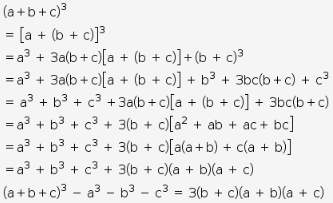# Prove (a+b+c)^3 - a^3 - b^3 - c^3 = 3(a+b) (b+c) (c+a)

Prove (a+b+c)3 - a3 - b3 - c3 = 3(a+b) (b+c) (c+a)